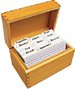# Section 10.3. Creating an Echo

[Page 315 (continued)]

### 10.3. Creating an Echo

Creating an echo effect is similar to the splicing method (Program 74 (page 300)) that we saw in the last chapter, but involves actually creating sounds that didn't exist before. We do that by actually adding wave forms. What we're doing here is adding samples from a delay number of samples away into the sound, but multiplied by 0.6 so that they're fainter.

##### Program 81. Make a Sound and a Single Echo of It (This item is displayed on pages 315 - 316 in the print version)`/** * Method to add an echo to a sound * @param delay the number of samples before the echo starts */ [Page 316]public void echo(int delay) { // make a copy of the original sound Sound s = new Sound(this.getFileName()); int value = 0; // loop from delay to end of sound for (int i = delay; i < this.getLength(); i++) { /* get the value back by delay samples from the * copy of the sound and make it fainter */ value = (int) (s.getSampleValueAt(i-delay) * 0.6); /* set the value at the current index to the sum * of the current value and the echo */ this.setSampleValueAt(i, this.getSampleValueAt(i) + value); } }`

[Page 317]

#### How it Works

The echo method takes a delay: the number of samples before the echo starts. Try this with different amounts of delay. With low values of delay, the echo will sound more like vibrato. Higher values (try 10,000 or 20,000) will give you a real echo.

• This method creates a copy of the current sound s. This is where we'll get the original, unadulterated samples for creating the echo. (You could try this without creating a copy to get some interesting layered echoes.)

• Next we declare a variable value to hold a value of a sample.

• Our loop starts with the index i being set to the passed delay and continues through the rest of the sound.

• The echoed sound is delay samples back, so i-delay is the sample we need. We multiply it by 0.6 to make it softer in volume.

• We then add the echoed sample to the current sample at i and set it in the current Sound object.

Try this method on sounds with words in them (Figure 10.3).

`> String fileName = FileChooser.getMediaPath("thisisatest.wav"); > Sound sound = new Sound(fileName); > sound.explore(); > sound.echo(20000); > sound.explore();`

##### Figure 10.3. The original "This is a test" sound (left), and the sound with an echo (right).#### 10.3.1. Creating Multiple Echoes

This method actually lets you set the number of echoes that you get. You can generate some amazing effects in this way.

##### Program 82. Creating Multiple Echoes (This item is displayed on pages 317 - 318 in the print version)`/** * Method to create multiple echoes of the current sound * @param delay the number of samples before the echo starts [Page 318] * @param numEchoes the number of echoes desired * @return a new sound with the echoes in it */ public Sound echo(int delay, int numEchoes) { int soundLength = this.getLength(); Sound echoSound = new Sound(numEchoes * delay + soundLength); int value = 0; int echoIndex = 0; int echoValue = 0; double echoAmplitude = 1; // to start // copy the original sound echoSound.splice(this,0,soundLength,0); /* loop starting with 1 to create the first echo at the * right place and end when = the number of echoes */ for (int echoCount = 1; echoCount <= numEchoes; echoCount++) { // decrease the volume (amplitude) of the echo echoAmplitude = echoAmplitude * 0.6; // echo the whole sound for (int i = 0; i < soundLength; i++) { echoIndex = i + (delay * echoCount); echoValue = (int) (this.getSampleValueAt(i) * echoAmplitude); echoSound.setSampleValueAt(echoIndex,echoValue + echoSound.getSampleValueAt(echoIndex)); } } return echoSound; }`

To try out this method, create a Sound object, and then invoke this method on the Sound object. Be sure to save the resulting Sound.

`> Sound sound = new Sound(FileChooser.getMediaPath("croak.wav")); > Sound echo = sound.echo(8000,5); > echo.play();`Introduction to Computing & Programming Algebra in Java(c) A Multimedia Approach
ISBN: N/A
EAN: N/A
Year: 2007
Pages: 191